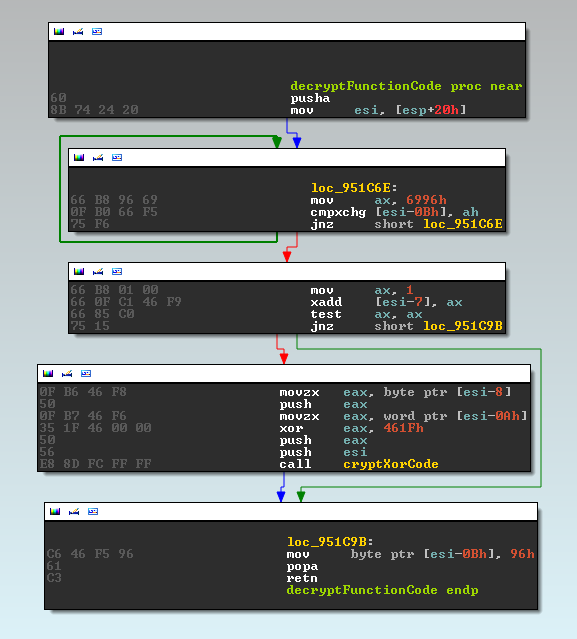## 18.08.2015

### Knowledge Fragment: Unwrapping Fobber

About two weeks ago I came across an interesting sample using an interesting anti-analysis pattern.
The anti-analysis technique can be best described as "runtime-only code decryption". This means prior to execution of a function, the code is decrypted, then executed and finally encrypted again, but with a different key.
Malwarebytes has already published an analysis on this family they called "Fobber".
However, in this blog post I wanted to share how to "unwrap" the sample's encrypted functions for easier analysis. There is also another blog post detailing how to work around the string encryption.

The sample and code related to this blog post can be found on bitbucket.

### Fobber's Function Encryption Scheme

First off, let's have a look how Fobber looks in memory, visualized by IDA's function analysis:IDA's first view on Fobber.

IDA only recognizes a handful of functions. Among these is the actual code decryption routine, as well as some code handling translating relevant addresses of the position independent code into absolute offsets.

Next, a closer look at how the on-demand decryption/encryption of functions works:The Fobber-encrypted function sub_95112A, starting with call to decryptFunctionCode.

We can see that function sub_95112A starts with a call to what I renamed "decryptFunctionCode":Fobber's on-demand decryption code for functions, revealing the parameter offsets neccessary for decryption.

This function does not make use of the stack, thus it is surrounded by a simple pushad/popad prologue/epilogue. We can see that some references are made relative to the return address (initially put into esi by copying from [esp+20h]):
• Field [esi-7] contains a flag indicating whether or not the function is already decrypted.
• Field [esi-8h] contains the single byte key for encryption, while
• field [esi-Ah] contains the length of the encrypted function, stored xor'ed with 0x461F.

The actual cryptXorCode takes those values as parameters and then loops over the encrypted function body, xor'ing with the current key and then updating the key by rotating 3bit and adding 0x53.Function for decrypting one function, given the neccessary parameters.

After decryption, our function makes a lot more sense and we can see the default function prologue (push ebp; mov ebp, esp) among other things.The decrypted equivalent of function sub_95112A, revealing some "real" code.

Also note the parameters:
• 0x951125 - key: 0x7B
• 0x951126 - length: 0x4629^0x461F -> 0x36 bytes
• 0x951128 - encryption flag: 0x01

So far so good. Now let's decrypt all of those functions automatically.

### Decrypt All The Things

First, we want to find our decryption function. For all Fobber samples I looked at, the regex r"\x60\x8B.\x24\x20\x66" was delivering unique results for locating the decryption function.

Next, we want to find all calls to this decryption function. For this we can use the regex r"\xE8" to find all potential "call rel_offset" instructions.
Then we just need to do some address math and check if the call destination (calculated as: image_base + call_origin + relative_call_offset + 5) is equal to the address of our decryption function.
Should this be the case, we can extract the parameters as described above and decrypt the code.

We then only need to exchange the respective bytes in our binary with the decrypted bytes. In the following code I also set the decryption flag and fix the function ending with a "retn" (0xC3) instruction to ease IDA's job of identifying functions afterwards. Otherwise, rinse/repeat until all functions are decrypted.

Code:

#!/usr/bin/env python
import re
import struct

def decrypt(buf, key):
decrypted = ""
for char in buf:
decrypted += chr(ord(char) ^ key)
# rotate 3 bits
key = ((key >> 3) | (key << (8 - 3))) & 0xFF
key = (key + 0x53) & 0xFF
return decrypted

def replace_bytes(buf, offset, bytes):
return buf[:offset] + bytes + buf[offset + len(bytes):]

def decrypt_all(binary, image_base):

# locate decryption function
decrypt_function_offset = re.search(r"\x60\x8B.\x24\x20\x66", binary).start()

# locate all calls to decryption function

regex_call = r"\xe8(?P<rel_call>.{4})"
for match in re.finditer(regex_call, binary):
call_origin = match.start()
packed_call = binary[call_origin + 1:call_origin + 1 + 4]
rel_call = struct.unpack("I", packed_call)
call_destination = (image_base + call_origin + rel_call + 5) & 0xFFFFFFFF
if call_destination == image_base + decrypt_function_offset:

# decrypt function and replace/fix

decrypted_flag = ord(binary[call_origin - 0x2])
if decrypted_flag == 0x0:
key = ord(binary[call_origin - 0x3])
size = struct.unpack("H", binary[call_origin - 0x5:call_origin - 0x3]) ^ 0x461F
buf = binary[call_origin + 0x5:call_origin + 0x5 + size]
decrypted_function = decrypt(buf, key)
binary = replace_bytes(binary, call_origin + 0x5, decrypted_function)
binary = replace_bytes(binary, call_origin + len(decrypted_function), "\xC3")
binary = replace_bytes(binary, call_origin - 0x2, "\x01")

return binary

[...]

IDA likes this this already better:IDA's view on a code-decrypted Fobber sample.

However, we are not quite done yet, as IDA still barfs on a couple of functions.

### Conclusion

After decrypting all functions, we can already start analyzing the sample effectively.
But we are not quite done yet, and the second post looks closer at the inline usage of encrypted strings.

sample used:
md5: 49974f869f8f5d32620685bc1818c957
1.